Teacher: Shannon
Customers Who Have Viewed This Course: 375
\$175.00

#### 0 Introduction 03:05

A quick description of the math unit and the thinking behind  the planning. This will give you a feel for my style and my expectations.

#### 1 Decimals Lesson 1 17:04

We will explore place value for whole numbers and decimals to practice comparing them.

#### 2 Decimals Lesson 1 practice review 05:33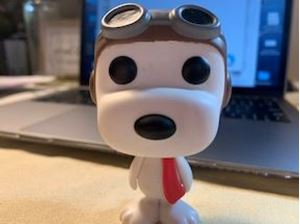Detailed explanation of each problem on the practice page.

#### 3 Decimals Lesson 2 11:14

This lesson looks at decimals as fractions and reviews different forms.

#### 4 Decimals Lesson 2 practice review 06:34Detailed explanation of practice page

#### 5 Decimals Lesson 3 14:50

We will talk about the real world application of adding and subtracting decimals.

#### 6 Decimals Lesson 3 practice review 08:37#### 7 Decimals Lesson 4 13:30

We will look at how we use rounding to estimate and practice our place value too.

#### 8 Decimals Lesson 4 practice review 09:58Detailed explanantion of each problem

#### 9 Decimals Quick Quiz 00:00

Let's review and see how you do with some simple and basic decimal work.

#### 10 Multiplication Lesson 1 10:02

I will walk you through multiplication terms and using zero when multiplying with 5's and 10's.

#### 11 Multiplication Lesson 1 practice review 03:45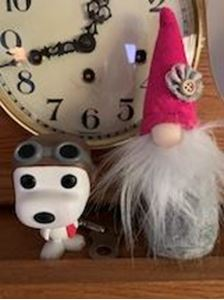Detailed review of each problem on the practice page.

#### 12 Multiplication Lesson 2 13:34

In this lesson we will multiply 2 digit numbers and introduce a method called place value boxes.

#### 13 Multiplication Lesson 2 practice review 12:18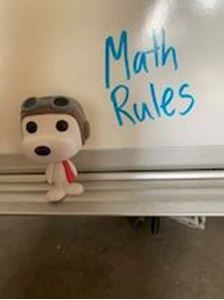Detailed explanation of each problem on the practice page.

#### 14 Multiplication Lesson 3 13:31

We will learn a great method for problem solving that will transfer to any operation. This will focus on multiplication.

#### 15 Multiplication Lesson 3 practice review 11:25We go over each story problem in detail to understand the process and answers.

#### 16 Multiplication Lesson 4 13:11

In this lesson we will use different methods to solve larger digit multiplication.

#### 17 Multiplication Lesson 4 practice review 07:11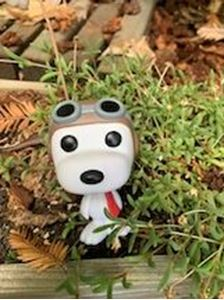Detailed answers to each problem on the practice page.

#### 18 Multiplication Lesson 5 10:22

This lesson builds on multiplication skills and we will multiply decimals.

#### 19 Multiplication Lesson 5 practice review 07:25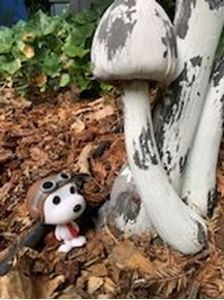#### 20 Multiplication Lesson 6 12:18

We practice all of our multiplication lessons here. Reviewing large digits and decimals with problem solving and practice the different strategies.

#### 21 Multiplication Lesson 6 practice review 11:58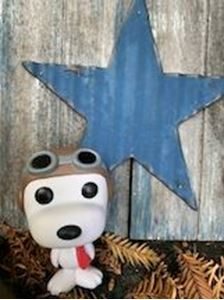Detailed explanation for each problem.

#### 22 Multiplication Quick Quiz 00:00

Multiply whole numbers and decimals.

#### 23 Division Lesson 1 13:25

This lesson is all about division with a single digit divisor and using remainders in answers. We will talk about terms to know and the steps needed for long division.

#### 24 Division Lesson 1 practice review 04:52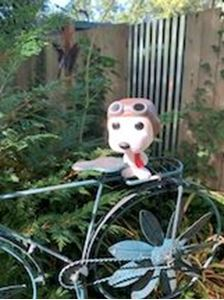We will go over the answers to each problem on the practice page.

#### 25 Division Lesson 2 14:09

Double digit divisors and fractional remainders in this lesson. We learn the fancy math words for all of the parts of a division problem and continue to work on long division skills.

#### 26 Division Lesson 2 practice review 14:22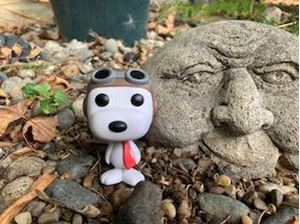Detailed answers to each problem on the practice page.

#### 27 Division Lesson 3 14:50

In this lesson we will discover what do you do with the remainder in a division problem? We will build on our divsion skillls while practicing multiplication and subtraction too. This lesson talks about being careful to read critically to solve word problems.

#### 28 Division Lesson 3 practice review 18:18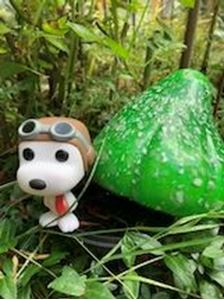Detailed explanation of each problem

#### 29 Division Lesson 4 12:21

In this lesson we will explore how money can be divided and how decimals are related.

#### 30 Division Lesson 4 practice review 13:48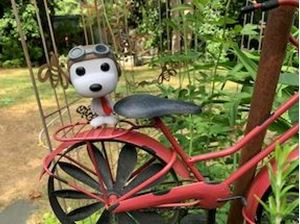We go over each problem so you can see how we get the answers.

#### 31 Division Lesson 5 13:08

We expolore dividing 2 decimals and using decimals as divisors with whole number dividends.

#### 32 Division Lesson 5 practice review 11:33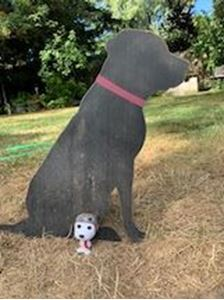Detailed explanations to the problems

#### 33 Division Quick Quiz 00:00

Division of whole numbers and decimals review.

#### 34 Fractions Lesson 1 18:24

We will review fraction basics and work on creating equivalent fractions. This is a great foundation lesson for further skills in fractions.

#### 35 Fractions Lesson 1 practice review 08:56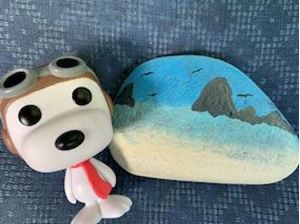Detailed examples of the practice page.

#### 36 Fractions Lesson 2 16:02

Building on our skills and compairing fractions by using common multiples. You will see why our warmup activities are so important. Making two fractions have the same denominator is an essential skill for later lessons.

#### 37 Fractions Lesson 2 practice review 12:32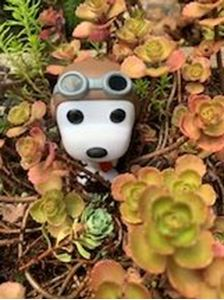Detailed explanation to the practice page.

#### 38 Fractions Lesson 3 10:23

We will turn mixed numbers into improper fractions: fractions that have whole numbers and fractions. This is a foundation skill you will need for later lessons.

#### 39 Fractions Lesson 3 practice review 04:12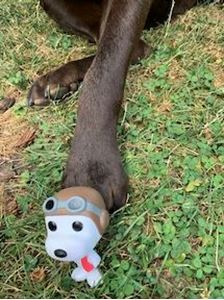#### 40 Fractions Lesson 4 10:18

Changing improper fractions to mixed numbers. This lesson is connected to lesson 3 and is an essential skill for the next lessons in this  fraction unit. We will build on our division skills as well as continue to use great math vocabulary.

#### 41 Fractions Lesson 4 practice review 07:08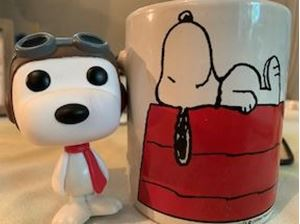A detailed explanation to each problem

#### 42 Fractions Lesson 5 16:11

This is all about how to use the GCF to simplify fractions.

#### 43 Fractions Lesson 5 practice review 05:56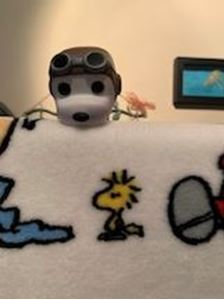Detailed examples for each problem

#### 44 Fractions Lesson 6 11:07

Addition and subtraction of fractions with the same denominator builds on our skills. We will practice finding common multiples and reducing our answers.

#### 45 Fractions Lesson 6 practice review 06:17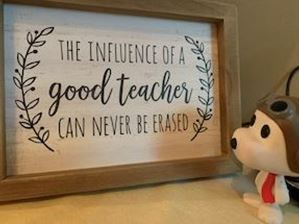#### 46 Fractions Lesson 7 12:58

In this lesson we use all our skills to make 2 fractions have the same denominator so we can add them and then simplify if needed. We will review equivalent fractions with our LCM/LCD.

#### 47 Fractions Lesson 7 practice review 12:24#### 48 Fractions Lesson 8 18:32

In this lesson we will learn how to borrow from a whole number of fraction to subtract. This is a great skill you will use in the next lessons. We will talk about 2 different strategies: remember how to make a top heavy fraction?

#### 49 Fractions Lesson 8 practice review 08:50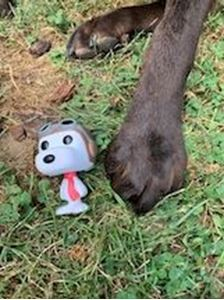#### 50 Fractions Lesson 9 14:26

Bringing all of our skills together to finish off this fractions unit to subtract fractions with unlike denominators. We will use our fancy borrowing skills to help us too.

#### 51 Fractions Lesson 9 practice review 09:37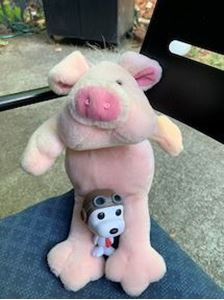#### 52 Fractions part 2 Lesson 1 12:02

This lesson introduces how to multiply fractions. We begin with unit fractions and whole numbers.

#### 53 Fractions part 2 Lesson 1 practice review 06:28#### 54 Fractions part 2 Lesson 2 11:23

In this lesson we move on and use what we know about unit fractions to help multiply non- unit fractions by whole numbers.

#### 55 Fractions part 2 Lesson 2 practice review 10:20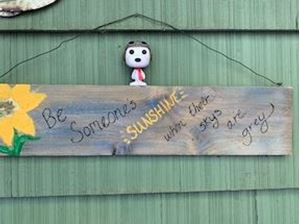#### 56 Fractions part 2 Lesson 3 15:47

This lesson teaches how to multiply two fractions.We practice division skills as we make sure we simplify all fractions. I also teach a fun trick called cross simplify which saves you some time.... if you cross simplify before you multiply then you will have a simplified answer.

#### 57 Fractions part 2 Lesson 3 practice review 11:04#### 58 Fractions part 2 Lesson 4 13:59

This lesson will build on our skills from a previous lesson about mixed numbers and improper fractions. We will multiply mixed numbers and practice reducing fractions too.

#### 59 Fractions part 2 Lesson 4 practice review 09:19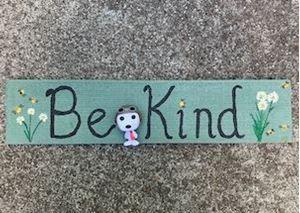#### 60 Fractions part 2 Lesson 5 14:08

This lesson will be all about division with fractional and whole number answers. We will learn some new VERY helpful formulas for dividing unit fractions and whole numbers.

#### 61 Fractions part 2 Lesson 5 practice review 04:51#### 62 Fractions part 2 Lesson 6 17:22

This lesson covers multiplication and divsion of fractions in story problems. We will talk about how to decide if you multiply or divide. We will practice using the formulas from previous lessons.

#### 63 Fractions part 2 Lesson 6 practice review 12:24#### 64 Pre-Algebra Lesson 1 15:01

In this lesson we will talk about important terms and vocabulary for algebra. This is a great introduction to algebra.

#### 65 Pre-Algebra Lesson 1 practice review 07:27#### 66 Pre-Algebra Lesson 2 15:47

Solving simple equations is like balancing a scale. This lesson will walk you through how to solve an equation and give you some creative ways to visualize them.

#### 67 Pre-Algebra Lesson 2 practice review 08:32#### 68 Pre-Algebra Lesson 3 12:35

This lesson walks you through how to take a story problem and write an algebraic equation then solve it.

#### 69 Pre-Algebra Lesson 3 practice review 06:33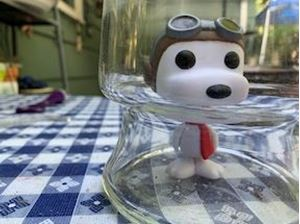#### 70 Pre-Algebra Lesson 4 16:09

This lesson builds on all of our skills and teaches how to write more complex equations and solve them.

#### 71 Pre-Algebra Lesson 4 practice review 14:39#### 72 Pre-Algebra Lesson 5 13:21

Introduction to PEMDAS, order of operations is an important skill. This lesson walks you through the process and helps you solve simple math sentences.

#### 73 Pre-Algebra Lesson 5 practice review 08:31#### 74 Pre-Algebra Lesson 6 14:09

This lesson reviews all of our skills: variables, equations and PEMDAS.

#### 75 Pre-Algebra Lesson 6 practice review 11:03#### 76 Coordinate Plane Lesson 1 12:08

This lesson introduces the coordinate plane. We work with ordered pairs in quadrant one and graph them as well as locate them.

#### 77 Coordinate Plane Lesson 1 practice review 05:41#### 78 Coordinate Plane Lesson 2 12:11

This lesson is about input and output with function tables. We will look at patterns that help us understand the rule used to fill in the table. This will lead to graphing those tables in our next lesson.

#### 79 Coordinate Plane Lesson 2 practice review 12:51#### 80 Coordinate Plane Lesson 3 11:33

This lesson wraps up coordinate plane with filling in charts for input and output then graphing them.

#### 81 Coordinate Plane Lesson 3 practice review 09:11#### 82 Measurement Distance Lesson 1 11:33

This lesson is about converting standard measurement for distance: inches, feet, yards and miles.

#### 83 Measurement Distance Lesson 1 practice review 07:10#### 84 Measurement capacity and weight Lesson 2 14:50

This lesson talks about standard measurements for capacity and weight.

#### 85 Measurement capacity and weight Lesson 2 practice review 12:21#### 86 Area and Perimeter Lesson 3 17:14

What is area and perimeter and how do you find it for rectangles and squares? Watch this lesson.

#### 87 Area and Perimeter Lesson 3 practice review 14:50#### 88 Polygons: Shapes Lesson 4 16:25

This lesson is about the different types of polygons. We look at angles and triangles and quadrilaterals.

#### 89 Polygons: Shapes Lesson 4 practice review 06:38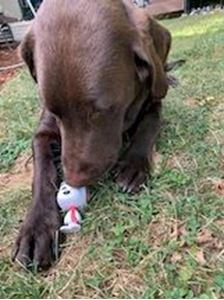#### 90 Volume and 3D shapes Lesson 5 18:08

This lesson walks you through the basics of prisms and pyramids. We also learn about cubic units for volume.

#### 91 Volume and 3D shapes Lesson 5 practice review 11:17#### 92 Measurement and Geometry QUIZ 00:00

Conversions, shapes and formulas quiz.

#### 93 Fun Math field trip 00:00

Open the attached document and see some real world examples of math. This is a fun family activity. Take a walk and look for math. If you can, take some photos and turn it into a presentation.

#### 94 Unit 1-3 Test 00:0020 story problems that help you review multiplication and division of whole numbers and decimals. The video to explain the answers is in the resource section.

#### 95 Unit 4 Test 00:00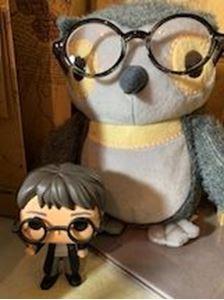This is a quiz to check for understanding of fractions: compare, simplify, addition and subtraction.

The video to explain the answers is in the resource section.

#### 96 Units 5-8 Test 00:00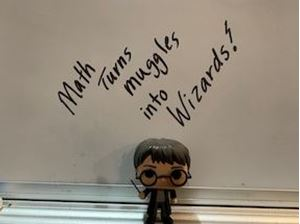This test will cover multiplication and division of fractions, pre- algebra, order of operations, coordinate points, measurement and geometry. The video explanation of answers is in the resource section.

Course Description: This is a fundamental maths skills course for 5th grade. It follows priority standards for math skills essential for moving on to a middle school level. There are 8 units with lessons that focus on essential skills broken down to smaller parts for mastery. Each lesson will have a video lesson with direct instruction. There will be example problems as well as an independent practice page. The lessons are under 20 minutes to help with focus and the practice is targeted to the specific skill in each lesson. There is a short quiz at the end of each unit to check for understanding.

This Course Includes:

- 8 units

- 44 video lessons

- 44 lesson activities/worksheets

- 44 practice problem review videos (I go over the details on how to solve each problem)

- Simple worksheets for each concept with counterpart answer sheets

- Online quick quizzes

- Combined unit Tests with video explanation of solutions

- Extra resources for notes and extended practice

Course Goal: Math is  an essential skill . I believe in finding a way to relate math to the real world. My goal is to teach real world skills and math that will help anyone find the confidence they need to learn math. I believe anyone can be successful in math if you practice and participate. I like to use some humor and fun when I teach math so get ready to have some fun while you learn.

Target Audience: This is a great course for a begining 5th grade math student as well as a great option for a 4th grader who may need a challenge here and there. The lessons are sequential and build on each other. Each concept has a 10-20 question practice page included that allows for ongoing assessment.

Requirements: Laptop, tablet or desktop computer can be used to watch lessons. All practice pages are in pdf format.  I suggest you have a spiral notebook and  use a pencil to take notes and work with me. I also encourage you to have your own smaller whitebord for practice problems and an easy way to work through longer or more complex work.

Course Oultine:

1.Decimals
L1 Place Value to Compare
L2 Decimals and Fractions (forms)
L3 Addition and Subtraction of decimals
L4 Rounding and estimation

Decimals QUIZ

2.Multiplication
L1 Multiples of 5 and 10
L2 Place Value sections  for double digits
L3 Problem Solving  Intro
L4 Larger Digit Multiplication
L5 Multiplying decimals
L6 Mixed review Multiplication

Multiplication QUIZ

3.Division
L1 Long division intro: single digit divisor
L2 Larger digit division: 2 digit divisor with fractional remainders
L3 Division problem solving: What to do with remainders
L4 Dividing money
L5 Dividing decimals

Division QUIZ

L1  Unit fractions and equivalent fractions
L2  How to Compare fractions
L3 Mixed Numbers to fractions
L4 Improper Fractions to Mixed Numbers
L5 Simplifying fractions
L6 Add and Subtractfractions with like denominators
L7 Adding fractions with unlike denominators
L8 How to borrow from a fraction and a whole number
L9 Subtraction with unlike denominators

5. Fractions  Multiply and divide
L1 Fractions of a whole number
L2 How to use a formula to multiply fractions
L3 Multiply 2 fractions and Simplify first
L4 Mixed number multiplication
L5 Dividing  fractions with Formulas
L6 Problem Solving with fractions

Fractions Quiz:  Mulitply and Divide

6. Pre- Algebra and Equations
L1 Equations and Algebraic terms
L2 Basic equations and how to solve them
L3 Writing equations and solving them
L4 Solving equations 2: balancing
L5 Intro to Order of Operations
L6 Evaluate equations with mixed operations.

Pre- Algebra Quiz

7.Graphing on the coordinate plane and functions
L1 Intro to coordinate plane quadrants : graphing points and locating points
L2 Looking for patterns and functions: input /output
L3  Graphing functions on the coordinate plane

8. Measurement/Geometry:area, perimeter,shapes and volume
L1 Standard Measurement distance
L2 Standard Measurement capacity and weight
L3 Area and Perimeter of simple  shapes
L4 Basic Shapes Geometry

L5 Volume and 3D shapes

Measurement and Geometry Quiz

Unit tests

• Teacher: Shannon
• Areas of expertise: 5th grade curriculum, technology and digital learning.
• Education: BA in Elementary Education, MAT Elementary Education.
• Interests: Insects, Hiking, dancing, dogs, and the beach.
• Skills: I am a great cook, I love to make jewelry and I am a super aunt and dog mom.
• Associations: State licensed teacher. Portland Oregon Rose Festival
• Issues I care about: I care about our environment, our future and education.

As predictable as it sounds, I truly believe children are our future and we need to invest in them. Education is the best way to open doors and I love learning with my students. Technology has revolutionized teaching as well as learning. Let's embrace it!

#### Test Preparation Document

Test Preparation Document

#### Digital Quizzes and Tests Answer Keys Document

Digital Quizzes and Tests Answer Keys Document

#### Mutliplication and Division Resources

Sometime the use of a multiplication chart can make the difference. Included here are more practice pages as well as some websites for extra practice.

#### Fraction Resources

There are lots of resources you can use to help with fractions.

#### Decimals Lesson 1 Key

The answers to the problems on the practice page

#### Multiplication Lesson 1 Key

Detailed explanations to each problem

#### Unit 1-3 Test

Detailed explanations for each problem.

#### Fractions Part 1 Test

The video shows how each problem was solved in detail. You can see an explanation for each problem.

#### Algebra Resources

Some helpful notes and a list of a great videos called Hands On Algebra.

#### Coordinate Plane Resources

Optional activities

#### Measurement and Geometry resources

Notes and an extra challenge page.

#### Unit 5-8 test

Detailed explanation for each problem

Customers who bought this item also boughtIn this complete science course students will utilize scientific skills of asking questions that will be answered through investigations, analysis, explanations, and identifying variables in an investigation. The course covers Physical Science, Earth Science, and Life Science. +
\$389.00 \$136.00Ready for some exciting chemistry labs? This course will build background knowledge of atoms, elements, periodic table, states of matter, phase changes and density with hands-on explorations and detailed video lessons. +
\$299.00